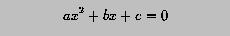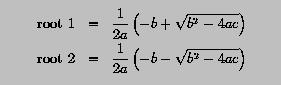# Quadratic Equation Solver - Revisited### Problem Statement

Given a quadratic equation as follows:if b*b-4*a*c is non-negative, the roots of the equation can be solved with the following formulae:Write a program to read in the coefficients a, b and c, and solve the equation. Note that a quadratic equation has repeated root if b*b-4.0*a*c is equal to zero.

However, if a is zero, the equation becomes a linear one whose only solution is -c/b if b is not zero. Otherwise, if b is zero, two cases are possible. First, if c is also zero, any number can be a solution because all three coefficients are zero. Otherwise, the equation c = 0 cannot have any solution.

This program should handle all cases.### Solution

```! ---------------------------------------------------
!   Solve  Ax^2 + Bx + C = 0 given B*B-4*A*C >= 0
!   Now, we are able to detect the following:
!    (1) unsolvable equation
!    (2) linear equation
!    (3) quadratic equation
!        (a) distinct real roots
!        (b) repeated root
!        (c) no real roots
! ---------------------------------------------------

PROGRAM  QuadraticEquation
IMPLICIT  NONE

REAL  :: a, b, c
REAL  :: d
REAL  :: root1, root2

!  read in the coefficients a, b and c

READ(*,*)  a, b, c
WRITE(*,*) 'a = ', a
WRITE(*,*) 'b = ', b
WRITE(*,*) 'c = ', c
WRITE(*,*)

IF (a == 0.0) THEN              ! could be a linear equation
IF (b == 0.0) THEN           ! the input becomes c = 0
IF (c == 0.0) THEN        ! all numbers are roots
WRITE(*,*)  'All numbers are roots'
ELSE                      ! unsolvable
WRITE(*,*)  'Unsolvable equation'
END IF
ELSE                         ! linear equation
WRITE(*,*)  'This is linear equation, root = ', -c/b
END IF
ELSE                            ! ok, we have a quadratic equation
d = b*b - 4.0*a*c
IF (d > 0.0) THEN            ! distinct roots?
d     = SQRT(d)
root1 = (-b + d)/(2.0*a)  ! first root
root2 = (-b - d)/(2.0*a)  ! second root
WRITE(*,*)  'Roots are ', root1, ' and ', root2
ELSE IF (d == 0.0) THEN      ! repeated roots?
WRITE(*,*)  'The repeated root is ', -b/(2.0*a)
ELSE                         ! complex roots
WRITE(*,*)  'There is no real roots!'
WRITE(*,*)  'Discriminant = ', d
END IF
END IF

END PROGRAM  QuadraticEquation
```
Click here to download this program.### Program Input and Output

Since we have been doing the quadratic part in several similar examples, the following concentrates on the order part of the program.
• If the input to the program consists of 0.0, 1.0 and 5.0, we have the following output. Since a is zero, this could be a linear equation. Since b=1.0, it is a linear equation 1.0*x + 5.0 = 0.0 with the only root -5.0/2.0=-5.0.
```0.0  1.0  5.0

a = 0.E+0
b = 1.
c = 5.

This is linear equation, root = -5.
```
• If the input to the program consists of 0.0, 0.0 and 0.0, we have the following output. Since all coefficients are zero, any number is a root.
```0.0  0.0  0.0

a = 0.E+0
b = 0.E+0
c = 0.E+0

All numbers are roots
```
• If the input to the program consists of 0.0, 0.0 and 4.0, we have the following output. Since the equation becomes 4.0 = 0.0, which is impossible. Therefore, this is not a solvable equation.
```0.0  0.0  4.0

a = 0.E+0
b = 0.E+0
c = 4.

Unsolvable equation
```### Discussion

Here is the box trick of this program. It is more complex than all previous versions of quadratic equation solvers.

Let us start with linear equations.

 a = 0 it could be a linear equation a quadratic equation

Since we have learned to do the quadratic part, we shall do the linear equation part first. To be a linear equation, b cannot be zero and this has to be addressed in the upper rectangle:

 a = 0 b = 0 need to know if c is zero the equation is linear b*x+c=0 a quadratic equation

If c is zero, then every number is a root and we have:

 a = 0 b = 0 c = 0 every number is a root this equation is not solvable the equation is linear b*x+c=0 a quadratic equation

To complete the bottom rectangle, let us take the box from previous example. The final result is:

 a = 0 b = 0 c = 0 every number is a root this equation is not solvable the equation is linear b*x+c=0 b*b - 4.0*a*c ? 0.0 > computes the real roots = computes the repeated root > no real root

The above program is written based on this logic.